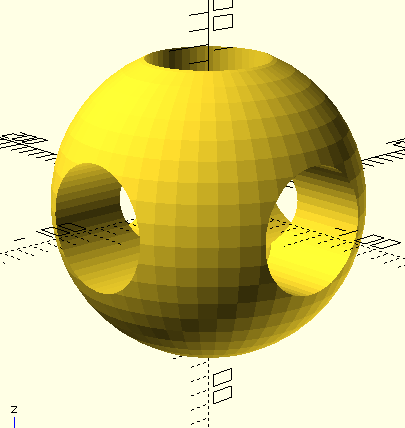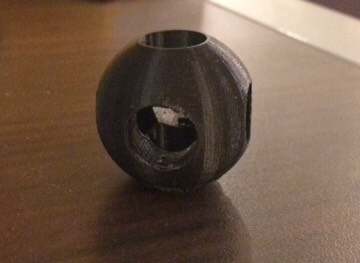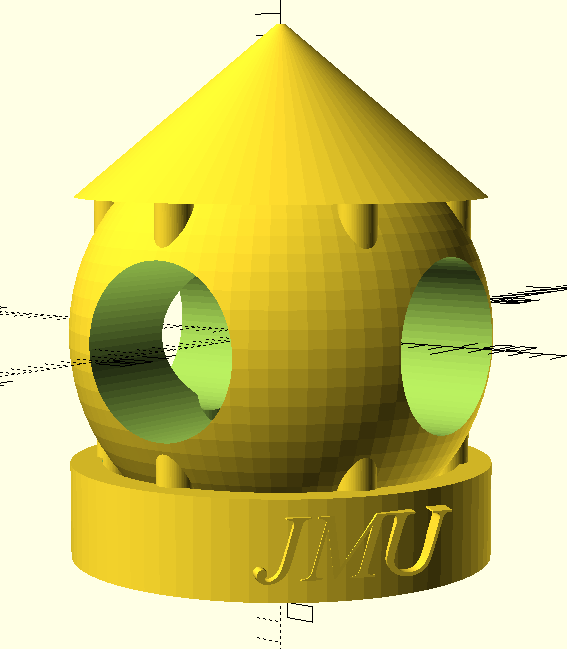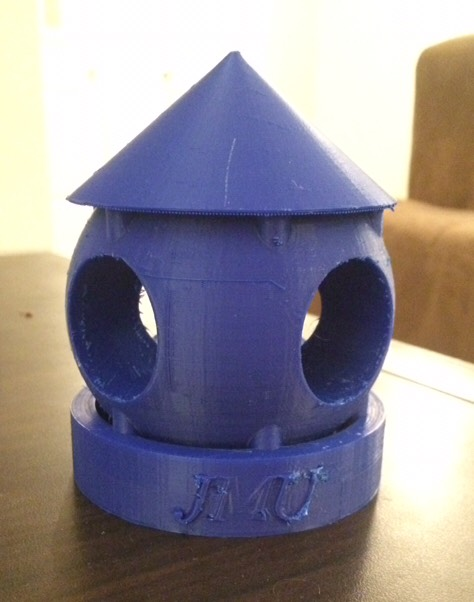# Mole Hole Cage

Working with OpenSCAD was really fun! During the fact that I’m planning on declaring a CIS minor, where you also have to write codes, I could already practice my skills for later on! To be honest, it did not take me that long to figure out how this program works; after listening to Mrs. Taalman and getting some help from the Cheat Sheet I already was ready to go. Compared to the other programs we used throughout the semester, I liked OpenSCAD the most.

To start off, I sat down and thought about what I could do when given the opportunity to create something by writing codes. After a short while, I decided to create a figure which I decided to call “Mole Hole Cage”. I checked out the OpenSCAD logo and thought it looked really awesome, which let me decide to create something similar, to always think back to this amazing class when seeing this certain logo.

The code I used reads as follow:

\$fn = 50;                                                                                                         //smoothen surface

cyr = 40;                                                                                                          // set radius of cylinder equal

module logo()                                                                                                 //defining my object (in this case the Mole Hole)

{

difference () {                                                                                                  //subtract all things after the first

sphere(100);                                                                                                    //base

cylinder (r=cyr, h=300, center=true);

rotate([90,0,0]) cylinder (r=cyr, h=300, center=true);                        //rotate to get another hole at 90 degrees on x-axis

rotate([0,90,0]) cylinder (r=cyr, h=300, center=true);                        //rotate to get another hole at 90 degrees on y-axis

}

}

logo();

This code resulted in a cylinder with holes in it! To understand the code better, I attached a picture of it below:The printed out version looks like this:However, in order to take my model to the next level, I then sat down and decided to add little extras. I was watching Prison Break and then thought of putting the “Ball” behind bars as well! So I wrote this code while getting some help from the OpenSCAD Cheat-Sheet and also a quick hint from Mrs. Taalman!

So, my final code for this project looks like this: (I highlighted the new stuff in green.)

cyr = 40; //set radius of cylinder equal
module logo()                                                                             //first figure (ball with holes)
{
difference () {
sphere(100);
cylinder (r=cyr, h=300, center=true);
rotate([90,0,0]) cylinder (r=cyr, h=300, center=true);             //rotate to get another hole at 90 degrees on x-axis
rotate([0,90,0]) cylinder (r=cyr, h=300, center=true);             //rotate to get another hole at 90 degrees on y-axis
}
}
logo();

\$fn = 75;                                                                                               //smoothen
{
translate([0,0,-105]) {                                                                       //alignment of “cage” figure to place ball in the middle
difference() {
cylinder(h = 45, r = 100);
translate([0,0,25]) cylinder(h = 50, r = 85);
translate([50,0,35]);
}
for (i = [0:6]) {                                                                                  //approximations of Real Numbers during calculation to determine lower part of the bars
echo(360*i/6, sin(360*i/6)*80, cos(360*i/6)*80);
translate([sin(360*i/6)*80, cos(360*i/6)*80,0])
cylinder(h = 65, r=9);
}

translate([0,0,170])                                             //use cylinder to cover the figure (roof)
cylinder(h = 80,r1=97, r2=2);
}
}
for (i = [0:6]) {                                                                                //approximations of Real Numbers during calculation to determine upper part of the bars
echo(360*i/6, sin(360*i/6)*80, cos(360*i/6)*80);
translate([sin(360*i/6)*80, cos(360*i/6)*80,40])
cylinder(h = 30, r=9);
}
rotate(50,[0,0,1])                                                    // rotates around
translate([-35,-90,-95])                                         // moves to centered and outside
rotate(91,[5,0,0])                                                    // stands up the letters
linear_extrude (height = 10)                               //use to transform 2D-Text into 3D-Figure
text (“JMU”,
size = 28, //how big should the word be
font = “TimesNewRoman:style=italic”,                                     //choose favourite font
halign = “0, bottom”,                                                                     //horizontal alignment
valign = “0, bottom”,                                                                     //vertical alignment
\$fn = 100);                                                                                      //smoothen the edges

This code causes my figure to look like this:The final, printed version can be seen below:In my opinion, it’s definitely a leveled-up version of my previous model. I added many more code elements for the new version, which also can be seen when taking a look at the model itself. It features more details, and it’s also representing the “JMU” logo, which was important to me. However, I wanted to “linear_extrude” my model, most likely because I think it is a really cool feature! Overall, I’m very satisfied with my final version/model and definitely will take the printed-out version home to give it a nice place in order to have a reminder to this really cool class!

Here you can find the link to my Thingiverse Model:

https://www.thingiverse.com/thing:2688912